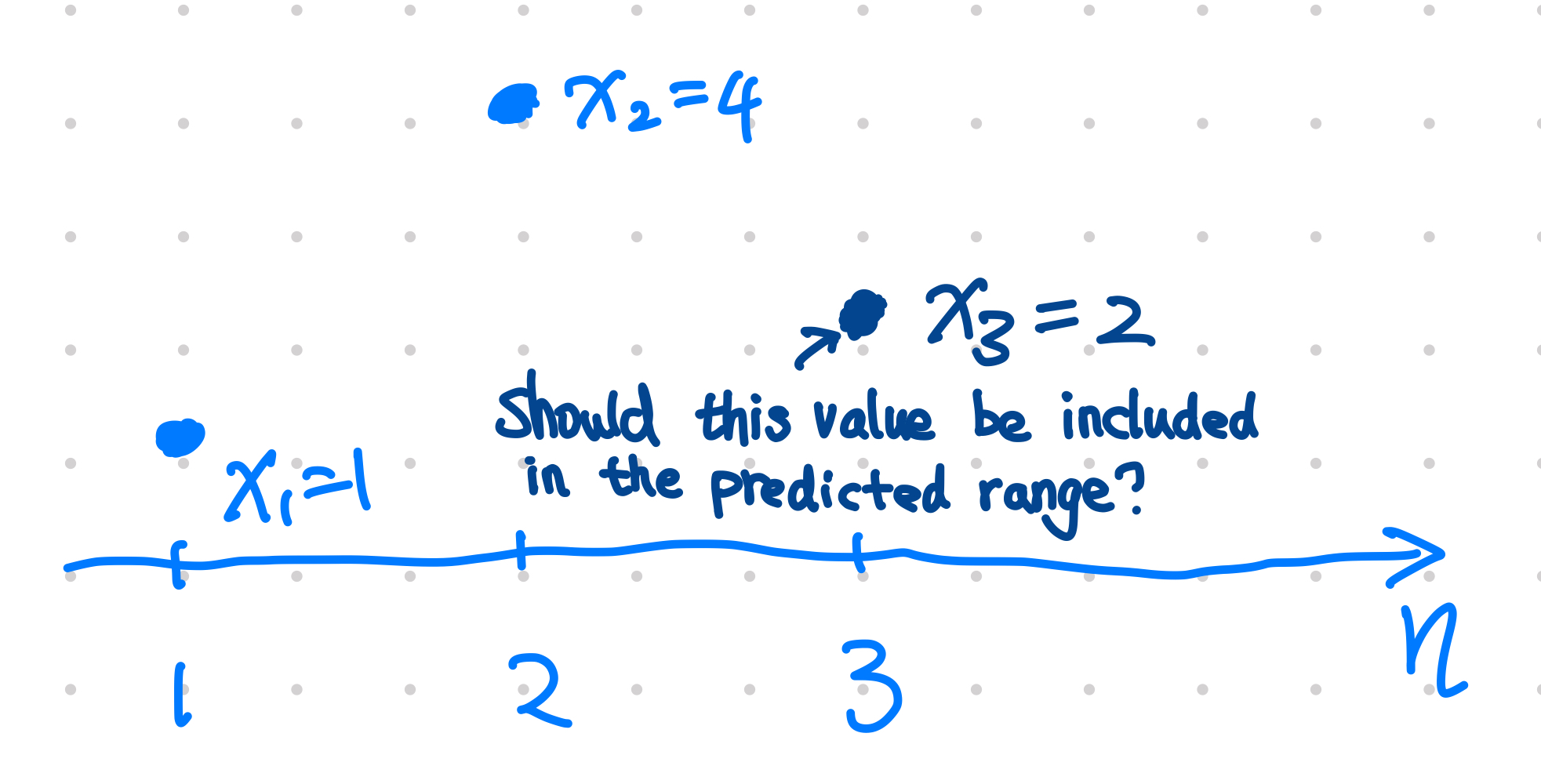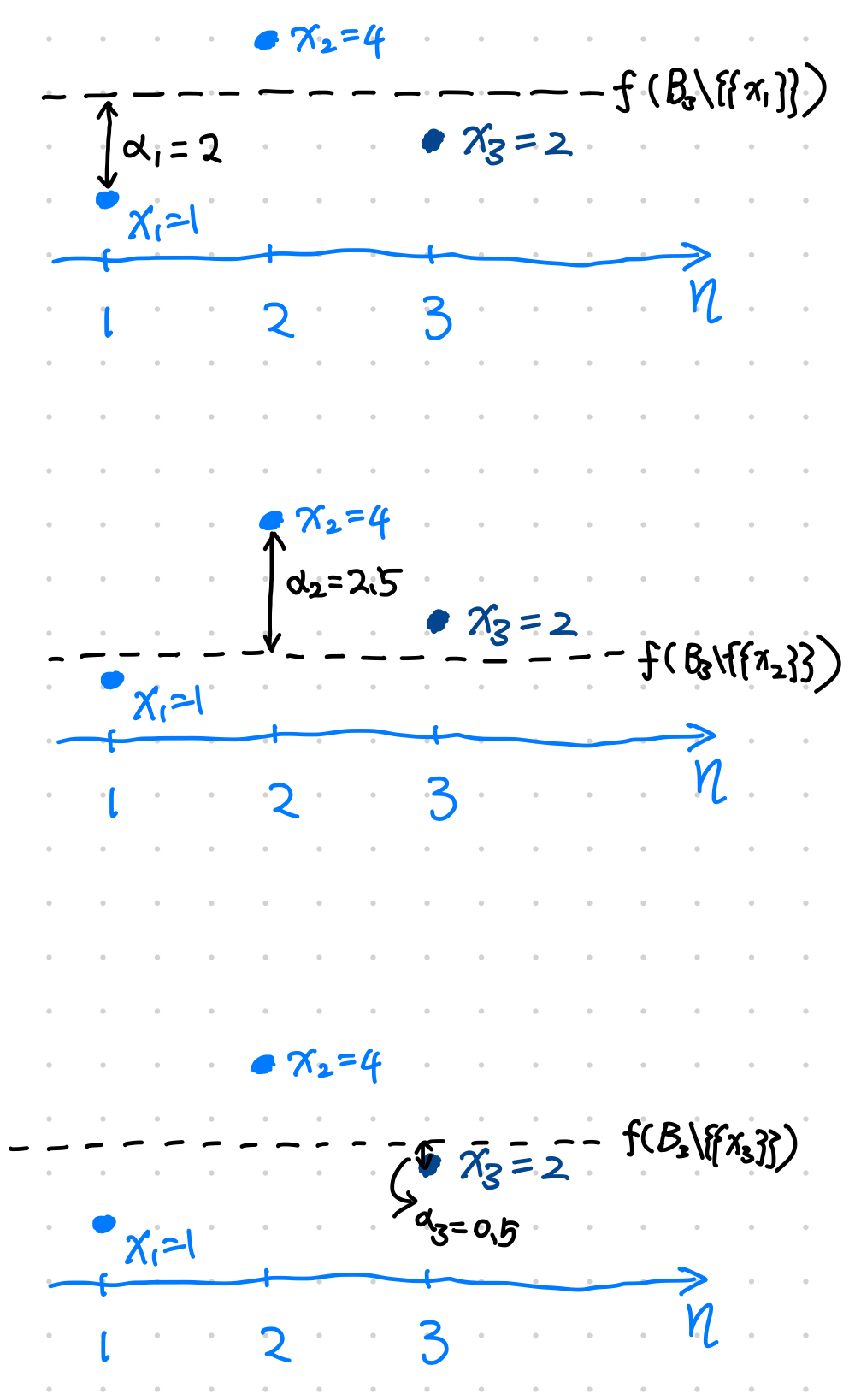Conformal prediction is a method to predict a consistent confidence interval in an on-line setting. The algorithms is following the framework, thus providing solid theoretical support for the predicted region.

on-line

An on-line setting is to predict continuously for sequentially incoming data points. For example, we predict $x_n$ for given data points $\{\!\{x_1, \cdots, x_{n-1}\}\!\}$. Then we predict $x_{n+1}$ using $\{\!\{x_1, \cdots, x_{n}\}\!\}$.

## Nonconformity Measure

For a new incoming data point $x$, and an existing of data points

$$B_n = \{\!\{x_1, \cdots, x_{n}\}\!\},$$

a nonconformity measure, $A(B_n, x)$, measures how different $x_n$ is from the existing multiset1.

A naive choice would be to reuse our model $x = f(B_n)$. Define a nonconformity measure

$$A(B_n, x) = d(f(B_n), x),$$

where $d$ is some kind of .

Choice of Nonconformity Measure

In principle, there are a lot of freedom in choosing the nonconformity measure. For example, as long as two nonconformity measures are monotonically related, the predicted regions are the same[^Shafer2007]. One could understand this using the following example.

## A Conformal Algorithm ExampleWe have two existing datapoints and try to decide whether the third data point $x_3$ should be included in the predicted region with $\alpha=0.05$ significance level.

Given two existing data points

$$B_3 = \{\{ x_1=1, x_2=4 \}\},$$

we will decide if $x_3=2$ should be included in our predicted region of significance level $\alpha=0.05$.

To be able to test if $x_3=2$ falls inside the predicted region, we simply take the average of data points for the point prediction, and we use L1 norm as the nonconformity measure, i.e.,

\begin{align} f(B_n) &= \frac{1}{n}\sum_i x_i \\ A(f(B_n), x) &= \lvert x - f(B_n) \rvert. \end{align}

With the model and nonconformity measure set, we can deicide whether the new data poin $x_3 = 2$ should be included in the predicted region of significance level $\alpha=0.05$.

For $i=1,2,3$,

1. calculate

$$f_i = f(B_3\{\{x_i\}\})$$

2. calculate the nonconformity measure

$$\alpha_i = A(f_i, x_i)$$

With all $\alpha_i$, count all the data points that leads to $\alpha_i\geq \alpha_3$, denoted as $N_{\alpha_i\geq\alpha_3}$, calculate the probability

$$p_x = \frac{N_{\alpha_i\geq\alpha_3}}{n}.$$Steps to decide whether to include $x_3=2$ in the predicted region.

Intuition using Extreme Cases

To get some intuition, let’s go to the extreme cases. If the new data point $x_3$ was not $2$ but $1e10$, we can easily see that $\alpha_1\sim\alpha_2\sim 1e10/2$ and $\alpha_3\sim 1e10$. That being said, $\alpha_3$ is always the largest and $p_x$ is minimized. If we have a lot of data points like $x_1$ and $x_2$, and suddenly there is a data point $x_n=1e10$, we have $p_x\to0$. This is an expected result as $1e10$ is so different.

This algorithm is basically a hypothesis test procedure using the 1.

## Requires Exchangeability

The algorithm assumes the data points are exchangeabile as we assume no statistical difference between

$$x_1, x_2, x_3, ..., x_n,$$

and

$$x_2, x_3, ..., x_n, x_1$$

and

$$x_1, x_3, ..., x_n, x_2$$

and all the possible permutations of this pattern. Obviously, i.i.d. data satisfies this condition. Note that i.i.d. is a more stringent condition1.

## Bonferroni Correction

For predictions involves multiple variable, we should consider the .

Planted: by ;

L Ma (2022). 'Conformal Prediction', Datumorphism, 04 April. Available at: https://datumorphism.leima.is/wiki/statistical-estimation/conformal-prediction/.